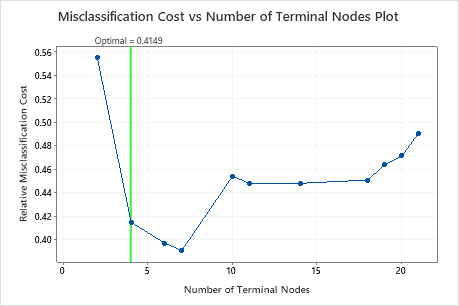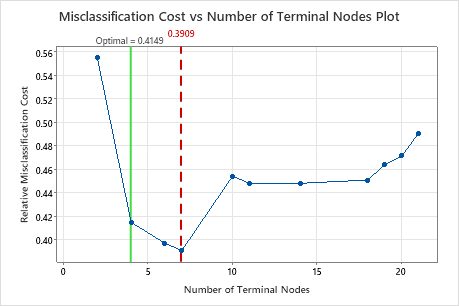# Select an alternative tree for CART® Classification

Run Stat > Predictive Analytics > CART® Classification. Click the Select an Alternative Tree button for the Misclassification cost vs. number of terminal nodes plot.

## Overview

By default, Minitab Statistical Software produces output for the smallest tree with a misclassification cost within 1 standard error of the smallest misclassification cost. Minitab lets you explore other trees from the sequence that led to the identification of the optimal tree. Typically, you select an alternative tree for one of the following two reasons:
• The optimal tree is part of a pattern where the misclassification costs decrease. One or more trees that have a few more nodes are part of the same pattern. Typically, you want to make predictions from a tree with as much prediction accuracy as possible. If the tree is simple enough, you can also use it to understand how each predictor variable affects the response values.
• The optimal tree is part of a pattern where the misclassification costs are relatively flat. One or more trees with similar model summary statistics have much fewer nodes than the optimal tree. Typically, a tree with fewer terminal nodes gives a clearer picture of how each predictor variable affects the response values. A smaller tree also makes it easier to identify a few target groups for further studies. If the difference in prediction accuracy for a smaller tree is negligible, you can also use the smaller tree to evaluate the relationships between the response and the predictor variables
For example, in the following plot, the tree with 4 nodes is the optimal tree. The next two larger trees are part of a pattern where the misclassification cost decreases.The 7-node tree has a misclassification cost that is less than the cost for the 4-node tree. Because the 7-node tree is similar in complexity, you can use the larger tree with its additional prediction accuracy to study the important variables and to make predictions.## Perform the analysis

Click Select an Alternative Tree in the output. A dialog box opens that shows the plot and a model summary table. The dialog box provides three ways to select alternative trees:
• Click a point on the graph.
• Click the arrow buttons under the model summary table to select a tree that is one tree larger or smaller than the current selection.
• Click a button to select a tree that is a common choice. When the analysis does not use validation, the buttons that refer to the standard error do not apply.
Min Cost
Select the tree with the minimum misclassification cost
1-SE Min Cost
Select the smallest tree that has a misclassification cost within one standard error of the minimum cost.
2-SE Min Cost
Select the smallest tree that has a misclassification cost within 2 standard errors of the minimum cost.
Best ROC
Select the tree with the greatest area under the ROC curve.

Click Create Tree to create and store results for an alternative tree that you choose. The selections for results and storage are the same as for the original tree. The graphs and tables for the alternative tree are in a new output tab. The stored columns are in the worksheet with the original data.

By using this site you agree to the use of cookies for analytics and personalized content.  Read our policy